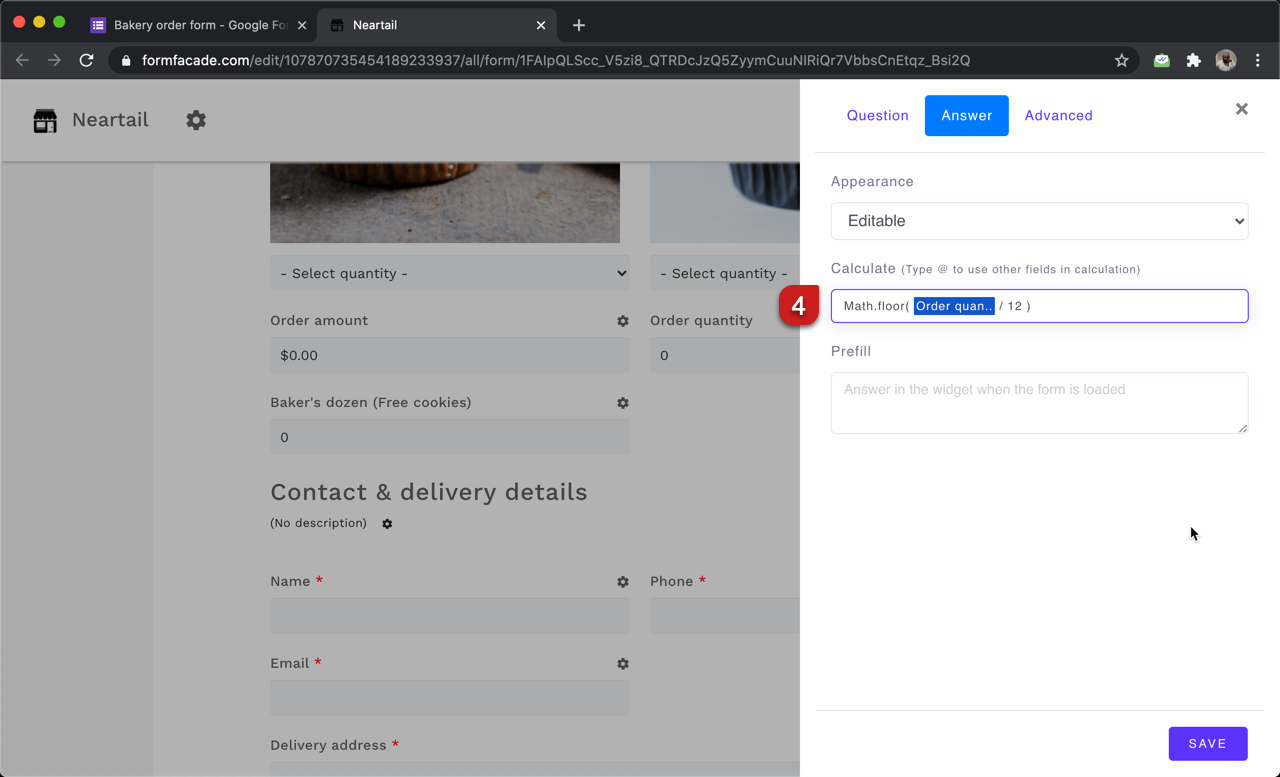# How to calculate the total quantity ordered by the users?

### You can use the QUANTITY function to calculate the order quantity for the products selected by the user. This will allow you to offer discounts, calculate shipping fees etc based on order quantity.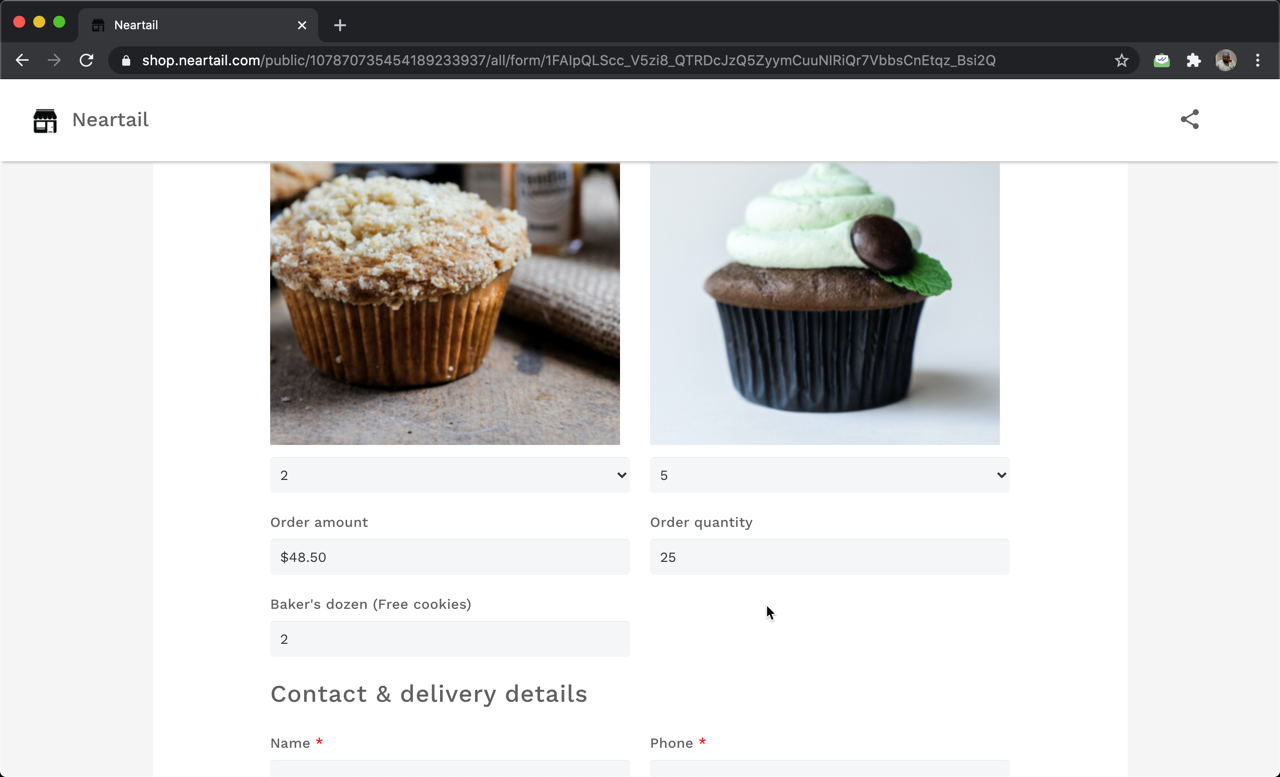#### How to calculate the order quantity?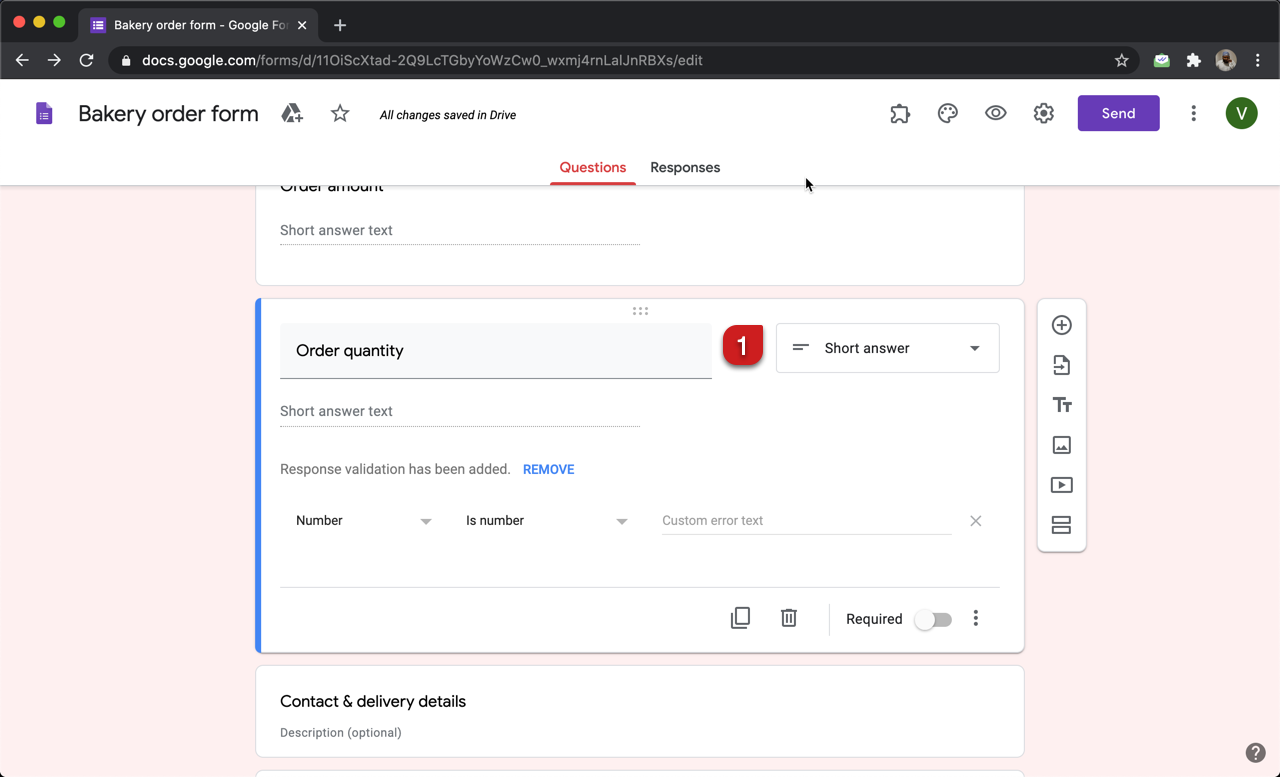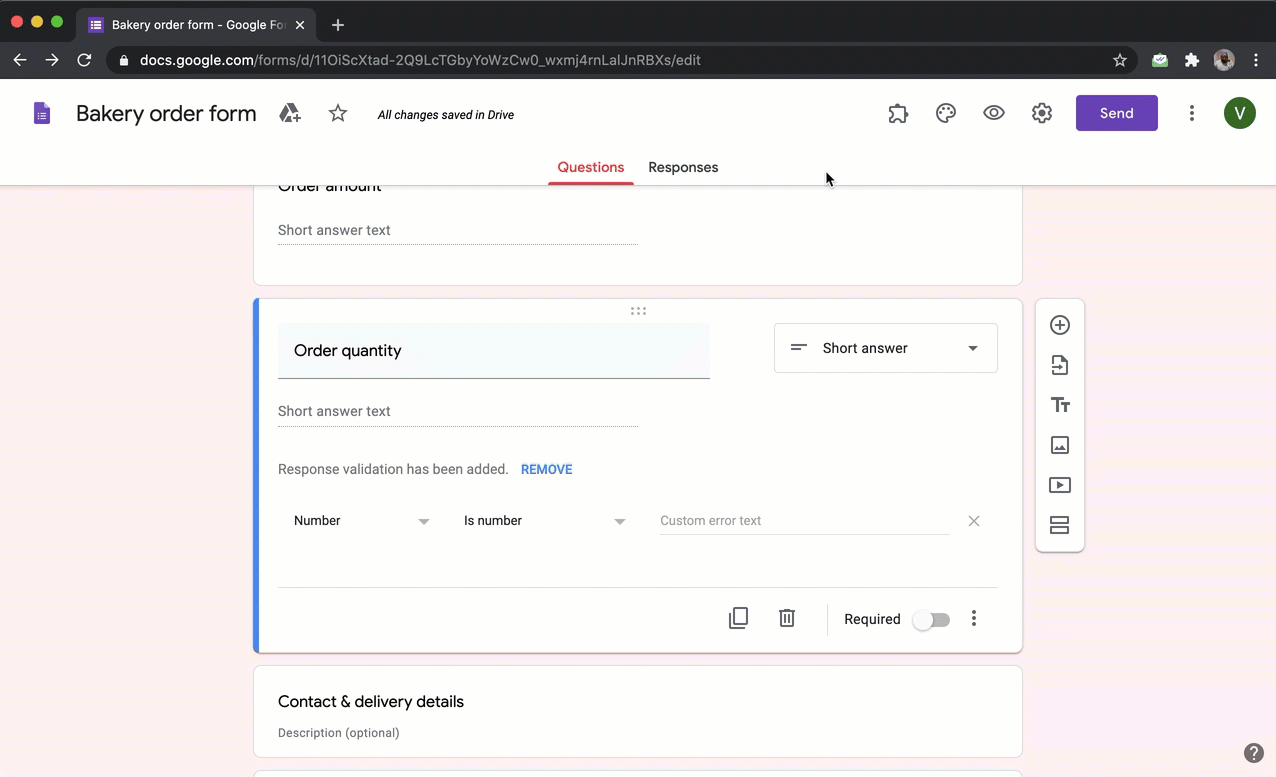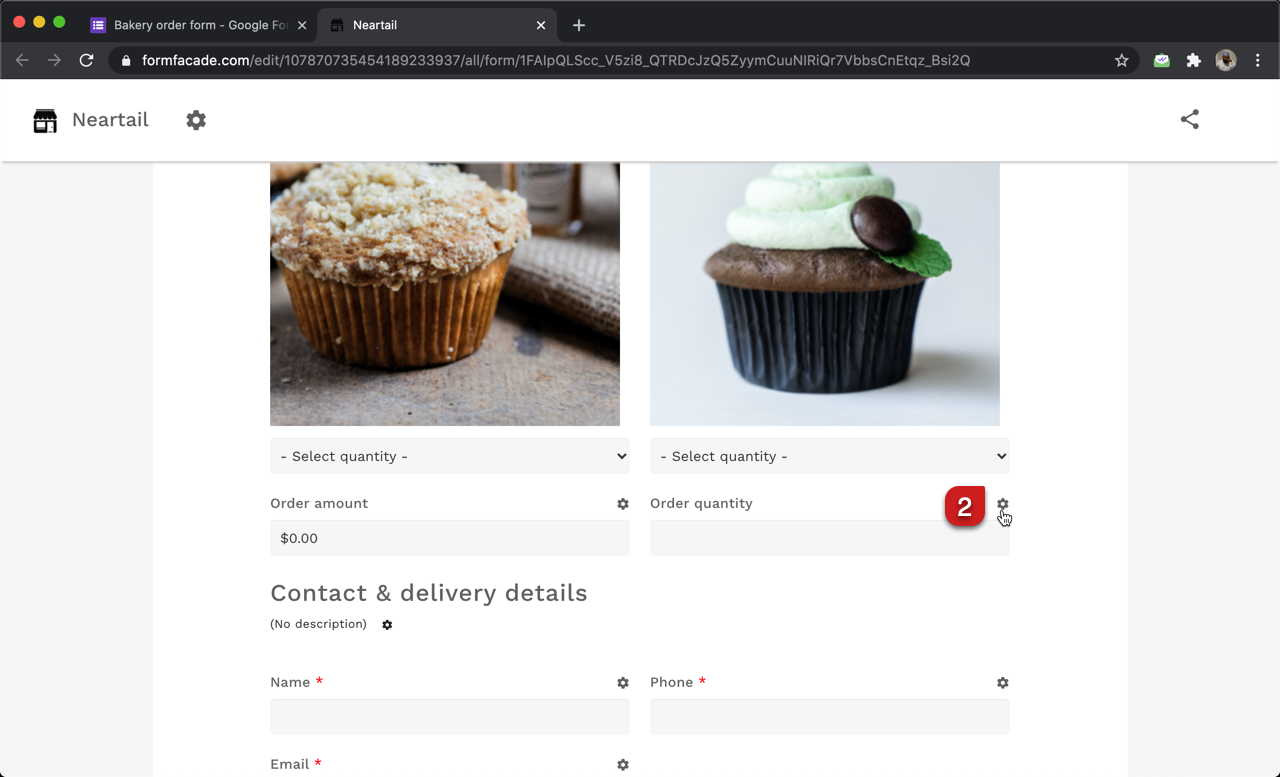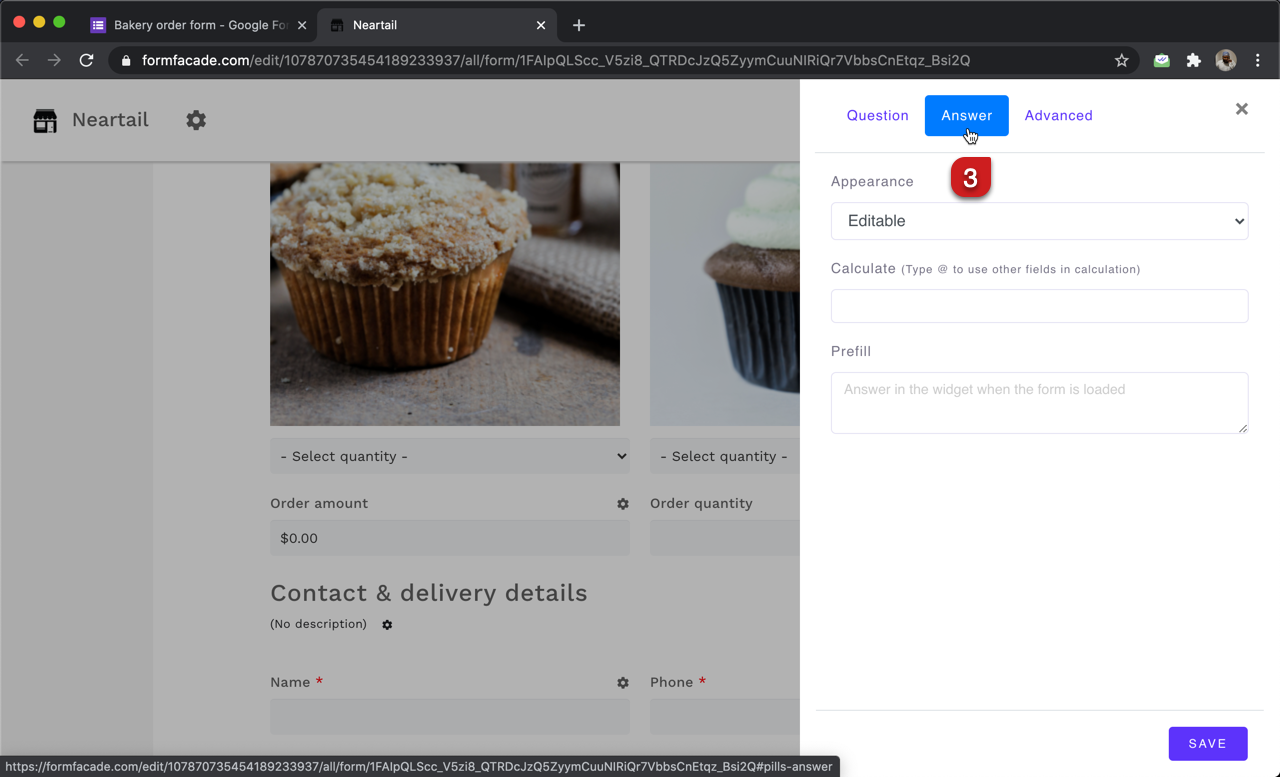#### Use QUANTITY function to calculate order quantity

You can use the QUANTITY function to calculate the order quantity. The syntax for the QUANTITY function is:

QUANTITY(currency)
where

• currency - currency symbol or text used to display the price of the products.

For example:

• If the price format is \$50 each, \$5.75 per kilo etc, the formula to calculate the order quantity is QUANTITY("\$")
• If the price format is €50 each, €5.75 per kilo etc, the formula to calculate the order quantity is QUANTITY("€")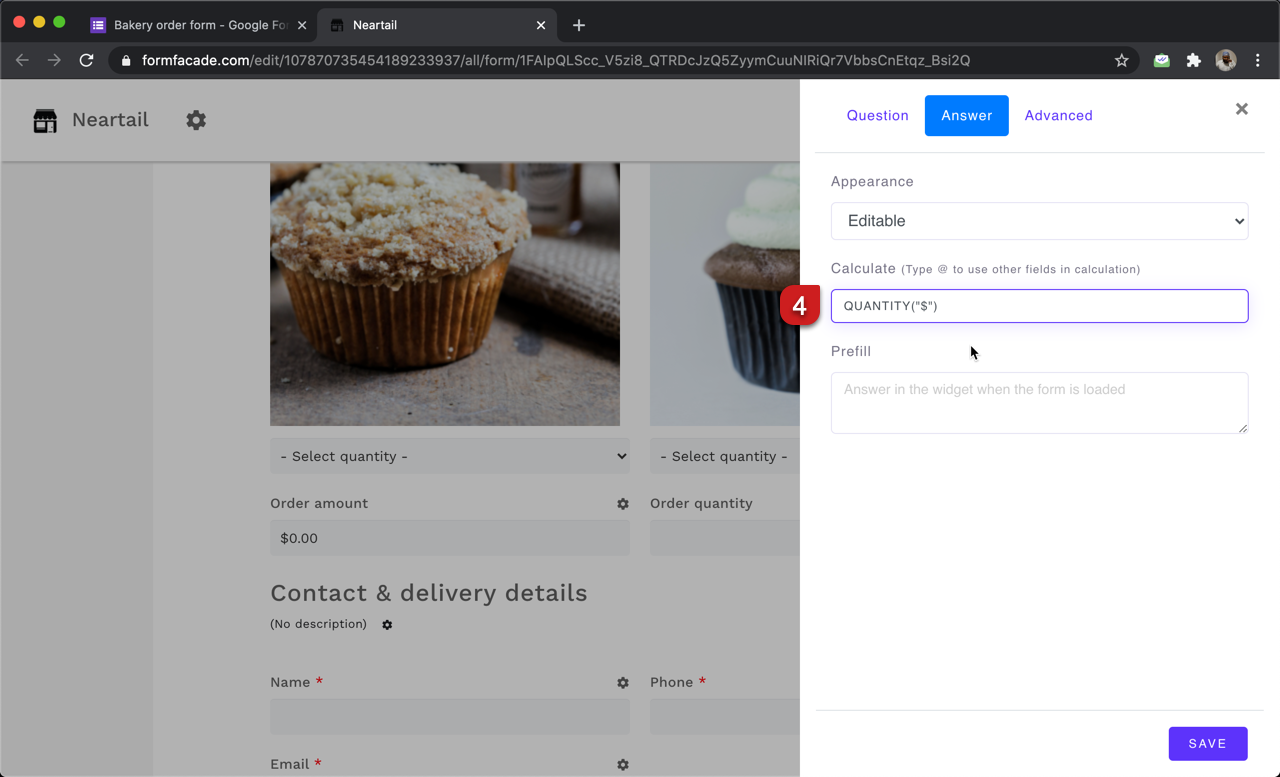#### Baker's dozen: How to offer a free cookie for every dozen?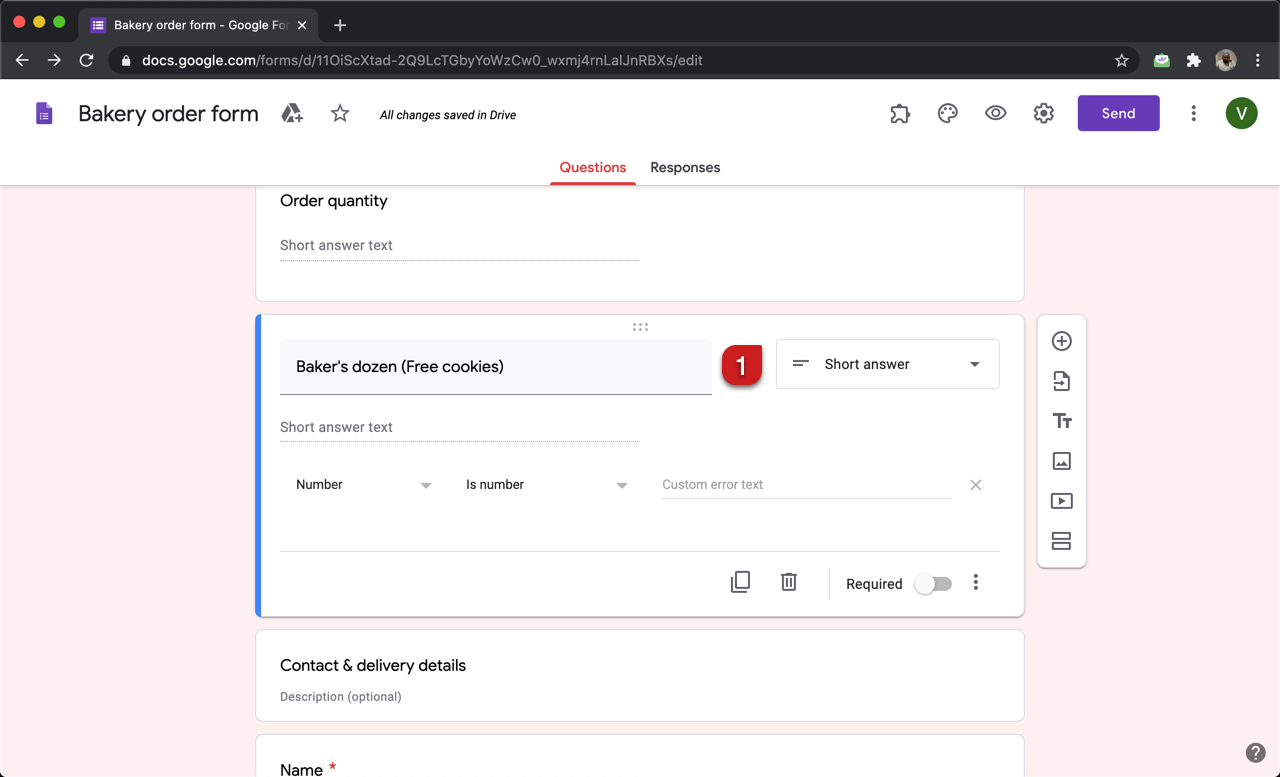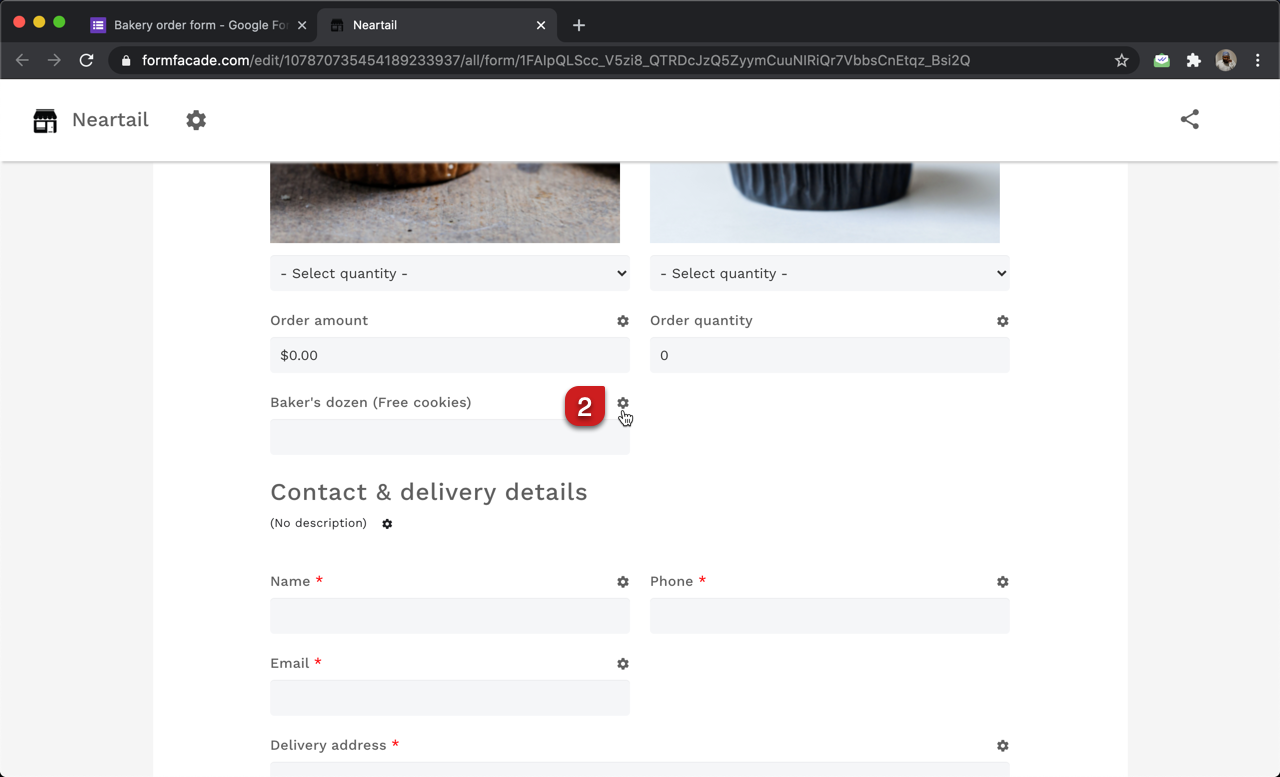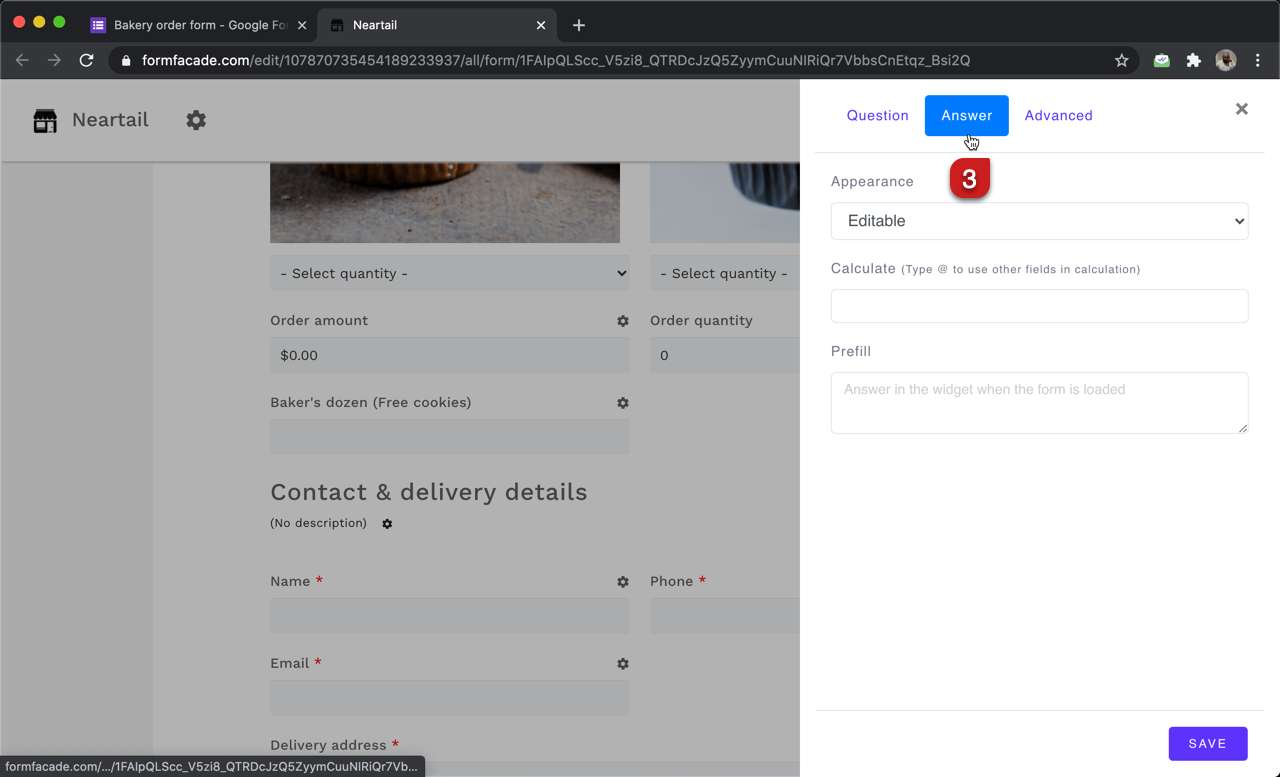#### Use Math.floor to calculate the number of dozens

Math.floor function returns the largest integer less than or equal to a given number. The syntax for Math.floor function is:

Math.floor(number)
where

• number - This could be a number or a calculation that returns a number.

Baker's dozen is 13. To offer a free cookie for every dozen that a user orders, we can calculate the number of dozens by dividing the order quantity by 12. Since we only need a round number, we can use the Math.floor function to calculate the exact number of free cookies.

Math.floor( Order quantity / 12 )